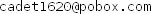# stack overflow Math bit 1ClassicListThreaded9 messagesOpen this post in threaded view
|

## stack overflow Math bit 1

 when I test Math class I get this error "stack overflow Math bit 1". all I did is that - declare static class named twoTothe and create it in the init function by size 15 according to A-instruction data represented by 15 bit and the most significant bit represents the sign of number -then initialize these entries of the array by i=0, n=1 while i<15 : twoTothe[i] = n; i = i+1; n= n+n; in bit function(int x, int i): i say if twoTothe[i] & x == 0 : return false ; else return true; that is all I did. may be there is something I don't understand causes the stack overflow exception.
Open this post in threaded view
|

## Re: stack overflow Math bit 1

 Administrator Stack overflows are usually caused by recursion gone wild.  For example (yours doesn't appear to) if bit() uses n=n*2 where '*' calls multiply() which calls bit() ... BOOM! Another common error in initializing the twoToThe Array (again, I don't see this in your code) is for the while loop to run wild after the 14th doubling because 16384 + 16384 = -32768 in 2's-complement numbers. If you want to, email me your Math.jack and I'll see if I can spot the problem. --MarkOpen this post in threaded view
|

## Re: stack overflow Math bit 1

 Thank you mark, you are right. a condition doesn't work correctly and that leads to overflow the stack I fixed it and pass the Math test but I notice this strange error. when I say var boolean b; let b = false; and then write if(b = false) I get this error a boolean value is illegal here. but i think according to the grammar of expression it is legal. ifstat : if '('expresssion')'...... expression : term (op term)* term : ....|.....|constantkey|....... constantkey : true| false| ...... op : ..|...|=|..... I ask why I get this error?
Open this post in threaded view
|

## Re: stack overflow Math bit 1

 Administrator hemasaad wrote var boolean b; let b = false; if(b = false) I get this error "A boolean value is illegal here". but i think according to the grammar of expression it is legal. ifstat : if '('expresssion')'...... expression : term (op term)* term : ....|.....|constantkey|....... constantkey : true| false| ...... op : ..|...|=|..... I ask why I get this error? You are correct that this is strictly legal by the Jack grammar. This is a case where the compiler is trying to be helpful and save you from a common problem. You should never compare to true and false. The safe way to write your test is: ```if (~b) ```--Mark
Open this post in threaded view
|

## Re: stack overflow Math bit 1

 I have another simple question in string class which is when the main.jack says let s = String.new(0); i note in the new method in string class when i print the maxLength it is 0 and that is right do s.dispose(); let s = String.new(6); code to append 5 chars but here the printed value isn't 6 but 3 and that is results in error because main will append 5 chars to string of length 3. i get 3 whatever the maxlength value passed by second string.new() I don't know why that occurs. may there is problem in dispose () ? all i do in dispose method is do Memory.dealloc(this); return;
Open this post in threaded view
|

## Re: stack overflow Math bit 1

 Administrator The normal implementation of String is a class with 3 fields: ```class String field int max_length; field int current_length; field Array data; // character data ```constructor String new(int maxLength) allocates the String object based on the number of fields, so all instances of String have fixed size = 3. The data Array is a separate allocation of size maxLength. String.dispose() needs to deallocate the data Array and then deallocate itself. --Mark
Open this post in threaded view
|

## Re: stack overflow Math bit 1

 I received a similar error "Stack overflow in Math.divide.12" when I executed the Math test. This is where Math.divide function recurses Math.divide. I showed a part of my code for Math.divide below. //a part of Math.divide function int divide(int x, int y){    var int q;    if (y>x){       return 0;    }    let q = Math.divide(x, y+y);    ... } I cannot see any issue but could you tell me the cause of the error? Ichiro